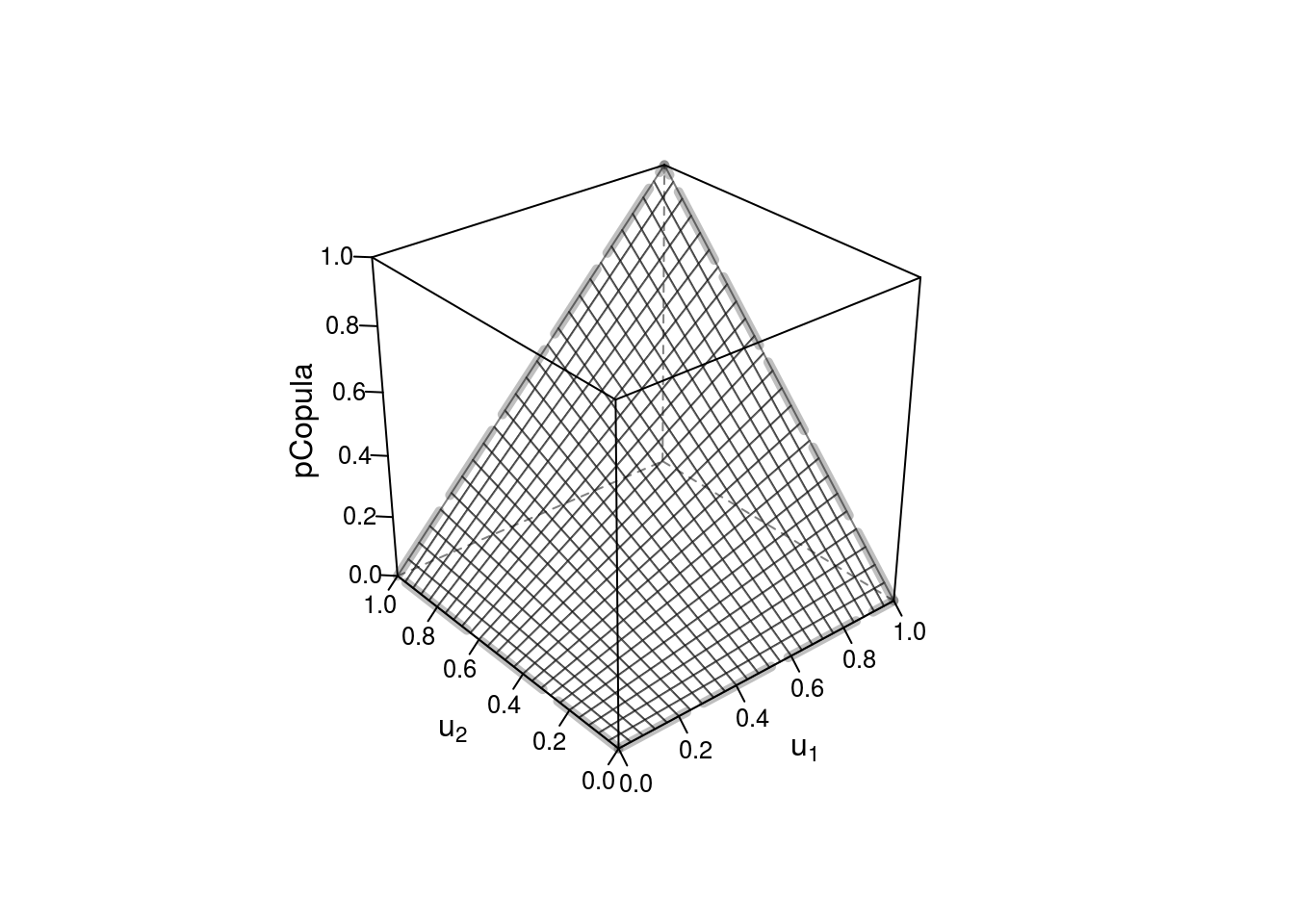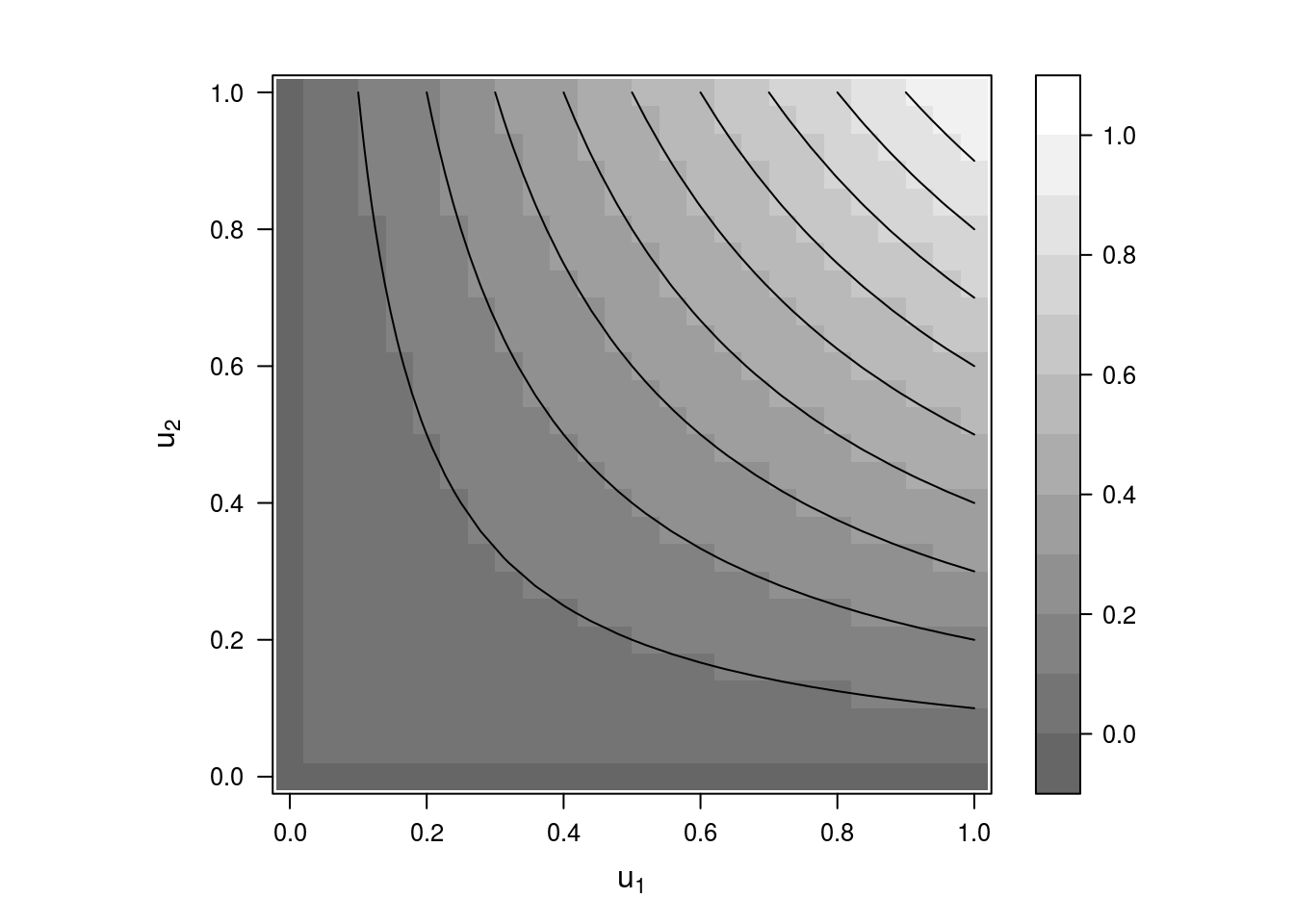## Chp 2

# load packages
libs<-c("copula")
invisible(lapply(libs, library, character.only = TRUE))

### Definition and characterization

#### Independence copula

d <- 2
ic <- indepCopula(dim = d)

set.seed(2008)
u <- runif(d) # a random point in the unit hypercube
(Pi <- pCopula(u, copula = ic)) # the value of the independence copula at u
##  0.3668219
stopifnot(all.equal(Pi, prod(u))) # check numerical equality of the samples
wireframe2  (ic, FUN = pCopula, # surface plot of the independence copula
col.4 = adjustcolor("black", alpha.f = 0.25))contourplot2(ic, FUN = pCopula) # contour plot of the independence copula